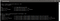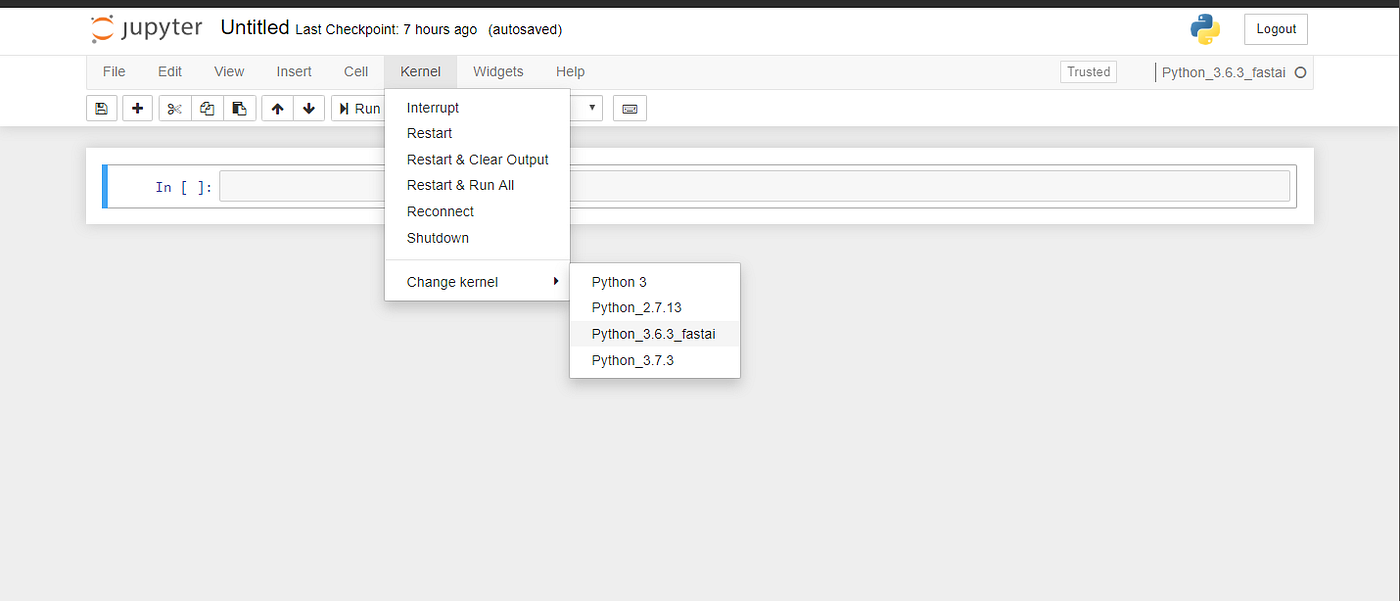# Beginner’s guide to setup and start coding in Jupyter Notebook in multiple versions of Python using Anaconda with Deep learning/NLP libraries

Hello there, I hope you find this easy and self-explanatory and gets you up ready to code in Python for machine learning or data science.

Jupyter notebook or what we call IPython Notebook is a widely used application in Data Science field for coding, testing, writing equations, plotting graphs. It is great practice to write a single line of code and run it rather than writing hundred lines and then running them as a whole. More on an incremental learning side jupyter provides you an easy go-to interface for experimenting with your code.

It is a server-client application that runs on any web browser of your choice without the need of internet access, on a localhost.

By the end of this, you will be able to setup your system ready for deep learning or NLP projects in a root or virtual environment depending upon your need!

# Contents

1. Install Anaconda
2. Using Jupyter Notebooks
4. Installing libraries or packages
5. Creating multiple virtual environments with different Python version for different ML needs
6. Installing Jupyter Notebook (kernel) in virtual environments
7. [OPTIONAL] Create an identical virtual environment in a different Windows machine

# Pre-requisites

• If you download Python 3.X version, it will install your ROOT environment (Base or default environment) with Python 3.X along with a set of many standard packages (like numpy, pandas, etc) that comes with Anaconda. Then you can create multiple virtual environments to work with different python versions or for different needs.
• If you download Python 2.X version, it will install your ROOT environment with Python 2.X along with a set of standard packages. Similarly you can create multiple virtual environments.
• So remember the version you install will determine which version of Python you are going to use more and extensively as your base. In this example, I am installing an older version of Anaconda Anaconda3–4.2.0-Windows-x86_64.exe found in https://repo.continuum.io/archive/

# Step 2. Install Anaconda

1. Click Next on the Welcome Screen.

2. Select a Destination Folder Path for installation.

3. If you choose a destination folder path containing spaces, it will show you a pop up warning, so it will be better to choose a path which does not contains spaces.

4. After choosing your installation path, Advanced Installation Options screen will show up, select both the options here.

5. Done.

# Step 3. Using Jupyter Notebooks

## Method 1:

Just select the Jupyter notebook Launch button. For the first time it will take some time, it opens a command prompt with an authentication token and then will open a Jupyter Dashboard on your default web browser.

## Method 2:

Here’s what the Jupyter Notebook Default Tree looks like:

The Jupyter dashboard opens in a Tree location by default which is generally `C:\Users\username\` mentioning various folders, files in that location. Now you can create a new notebook by clicking NEW located in top-right which gives you a drop-down mentioning your installed python version.

This opens a jupyter notebook. Voila! :)

Some useful shortcuts to get started

Press Escape key and then press

D — Delete a cell

M — Convert a cell into Markdown (text)

A — Add a cell below current cell

B — Add a cell above current cell

Shift+Enter — To execute (Run)

TIP : You can fully customize your notebook like the color, background, etc by editing the jupyter_notebook_config file.

# Step 4. Customizing your Jupyter Notebook

1) Open “Anaconda Prompt” and type `jupyter notebook --generate-config`

2) You find the file in `C:\Users\username\.jupyter\jupyter_notebook_config.py`

3) Find this line, `#c.NotebookApp.notebook_dir = ''` and change it to a directory you wish the dashboard to open at, like `c.NotebookApp.notebook_dir = 'c:\Your Dir'`

4) Then go to the shortcut of Jupyter Notebook generally located in `C:\Users\User_name\AppData\Roaming\Microsoft\Windows\Start Menu\Programs\Anaconda3 (64-bit)` and put it somewhere you will often use to open Jupyter Notebooks. Opening this shortcut will only enable this.

5) Hit the right click and select`Properties`

6) In the `Target` field, remove `%USERPROFILE%` in the end.

7) Then in the `Start in` field, type the same directory you just typed above `c:\Your Dir`

8) Now, Use this shortcut to open Jupyter Dashboard.

9) Done!

Now it’ll open the dashboard in your desired directory.

# Step 5. Installing libraries or packages

`(base) \$:>pip install pandas`

You can also install multiple libraries at once, mentioning their versions too!

`(base) \$:>pip install pandas==0.21.0 numpy==1.16.0`

# Step 6. Creating a virtual environment

Python applications will often use packages and modules that don’t come as part of the standard library. Applications will sometimes need a specific version of a library, or a different Python version.This means it may not be possible for one Python installation to meet the requirements of every application.

If application A needs version 1 of Python or of a particular module but application B needs version 2, then the requirements are in conflict and installing either version 1 or 2 will leave one application unable to run.

The solution for this problem is to create a virtual environment, a self-contained directory tree that contains a Python installation for a particular version of Python, plus a number of additional packages.Image shows a Base environment and other three virtual environments setup. You can also see the Jupyter Notebooks linked to each env.

## Virtual Environment Setup:

We have already installed Python 3.5.6 as Base environment. Now we will create three virtual environments (env) for different needs.

1. The first env is Python_2.7.13 which will be having Python version 2.7.13 with some basic libraries. For Natural Language Processing (NLP) tasks we will use NLTK library.
2. The second env is Python_3.6.3 which will be having Python version 3.6.3 with some basic libraries. For Deep Learning (DL) tasks we will use Keras (on top of Tensorflow) and for NLP we will have Spacy library.
3. The third env is Python_3.7.3 which will be having Python version 3.7.3 with some basic libraries. For Deep Learning we will use Pytorch and for NLP we will use fastai library.

NLP — There are many open-source libariees you can use like NLTK, Gensim, Spacy.

DL — There are many platforms depending upon various factors which makes each of them unique like Keras, Tensorflow, Pytorch, etc.

## PART 1: Virtual Environment “Python_2.7.13”

`conda create -n <ENV_NAME> python=<VERSION> <LIBRARY1> <LIBRARY2>`

like in this case:

`(base) \$:>conda create -n Python_2.7.13 python=2.7.13 numpy pandas scikit-learn nltk notebook ipykernel`

You can also install your env in the desired location (e.g. D:\Python\envs) instead of the default location which is `C:\ProgramData\Anaconda3\envs` like this:

`(base) \$:>conda create --prefix=D:\Python\envs\Python_2.7.13 python=2.7.13 numpy pandas scikit-learn nltk notebook ipykernel`

and then add the desired location path in conda config file like this:

`(base) \$:>conda config --append envs_dirs D:\Python\envs`

2. Activate your env: `(base) \$:>activate Python_2.7.13`

3. Once activated, you can install jupyter notebook and register it on kernelspec (to use all notebook kernels at one place) in one shot:-

`python -m ipykernel install --user --name <NAME> --display-name <DISPLAY NAME ON JUPYTER KERNEL LIST>`

`(Python_2.7.13) \$:>python -m ipykernel install --user --name Python_2.7.13_Notebook --display-name "Python_2.7.13"`

4. Now you can also install other libraries using PIP

`(Python_2.7.13) \$:>pip install seaborn`

5. Now just type `(Python_2.7.13) \$:>jupyter notebook `to launch jupyter notebook.

## PART 2: Virtual Environment “Python_3.6.3”

`(base) \$:>conda create --prefix=D:\Python\envs\Python_3.6.3 python=3.6.3 numpy pandas scikit-learn nltk notebook ipykernel`

2. Activate your env: `(base) \$:>activate Python_3.6.3`

3. Install pytorch and fastai libraries:

`(Python_3.6.3) \$:>conda install fastai pytorch=1.0.0 -c fastai -c pytorch -c conda-forge`

4. Install jupyter notebook

`(Python_3.6.3) \$:>python -m ipykernel install --user --name Python_3.6.3_Notebook --display-name "Python_3.6.3_fastai"`

5. Enter`(Python_3.6.3) \$:>jupyter notebook `to launch jupyter notebook.

## PART 3: Virtual Environment “Python_3.7.3”

`(base) \$:>conda create --prefix=D:\Python\envs\Python_3.7.3 python=3.7.3 numpy pandas scikit-learn nltk notebook ipykernel`

2. Activate your env: `(base) \$:>activate Python_3.7.3`

3. Now install Keras and Spacy libraries using PIP

`(Python_3.7.3) \$:>pip install keras(Python_3.7.3) \$:>pip install spacy`

`# Small Model (37 MB)(Python_3.7.3) \$:>python -m spacy download en_core_web_sm# Medium Model (120 MB)(Python_3.7.3) \$:>python -m spacy download en_core_web_md# Large Model (838 MB)(Python_3.7.3) \$:>python -m spacy download en_core_web_lg# Can load any of the above models using:> import spacy> nlp = spacy.load("en_core_web_md") # for using medium models`

4. Install jupyter notebook

`(Python_3.7.3) \$:>python -m ipykernel install --user --name Python_3.7.3_Notebook --display-name "Python_3.7.3"`

5. Enter`(Python_3.7.3) \$:>jupyter notebook `to launch jupyter notebook.

You can check the installed virtual environments using:

`(base) \$:>conda env list`

You can also check the installed kernels in Jupyter Notebook using:

`(base) \$:>jupyter kernelspec list`

In the above image, the left column are the names of Jupyter Notebook Kernels (the display name on screen may be different as specified while installing) and the right column are the location of these kernels.

The final Jupyter Notebook with multiple kernels looks something like this:You change Python Version by simply selecting the right kernel in ‘Change Kernel’ option.

# 8. Optional — Create an identical virtual environment in a different Windows machine

## STEPS…

`(base) \$:>conda activate my_ENV(my_ENV) \$:>conda env export > environment.yml(my_ENV) \$:>pip freeze > requirements.txt`

The issue in doing this way is, sometimes, the environment.yml file contains old or initial python package version numbers which creates an implementation error in a second machine. To prevent this, a workaround can be to create your own environment.yml file using requirements.txt, like mine below. (Just put all the requirement-packages under pip in yml file)

`environment.ymlname: python_3.5.6_stablechannels:  - defaultsdependencies:  - pip=19.0.0  - python=3.5.6  - pip:    - absl-py==0.7.1    - asn1crypto==0.24.0    - astor==0.8.0    - astroid==1.4.7    - atomicwrites==1.3.0    - attrs==19.1.0    - Babel==2.3.4    - backcall==0.1.0    - backports.weakref==1.0rc1    - beautifulsoup4==4.5.1    - bert-tensorflow==1.0.1    - bleach==3.1.0    - blis==0.2.4    - boto==2.49.0    - boto3==1.9.202    - botocore==1.12.202    - bs4==0.0.1    - bz2file==0.98    - cffi==1.12.3    - chardet==3.0.4    - Click==7.0    - colorama==0.3.9    - cryptography==2.7    - cycler==0.10.0    - cymem==2.0.2    - Cython==0.29.13    - datashape==0.5.2    - decorator==4.4.0    - defusedxml==0.6.0    - dill==0.3.0    - docutils==0.14    - et-xmlfile==1.0.1    - extract-msg==0.23.1    - Flask==1.1.1    - Flask-Cors==2.1.2    - future==0.17.1    - gast==0.2.2    - gensim==3.8.0    - grpcio==1.22.0    - gunicorn==19.9.0    - h5py==2.9.0    - html5lib==0.9999999    - idna==2.8    - imageio==2.5.0    - IMAPClient==2.1.0    - imbalanced-learn==0.5.0    - importlib-metadata==0.19    - ipaddress==1.0.22    - ipykernel==5.1.1    - ipython==7.7.0    - ipython-genutils==0.2.0    - itsdangerous==1.1.0    - jdcal==1.4.1    - jedi==0.14.1    - Jinja2==2.10.1    - jmespath==0.9.4    - joblib==0.13.2    - jsonschema==3.0.1    - jupyter-client==5.3.1    - jupyter-core==4.5.0    - Keras==2.2.4    - Keras-Applications==1.0.8    - Keras-Preprocessing==1.1.0    - kiwisolver==1.1.0    - lazy-object-proxy==1.4.1    - mail-parser==3.9.3    - Markdown==3.1.1    - MarkupSafe==1.1.1    - matplotlib==3.0.3    - mistune==0.8.4    - more-itertools==7.2.0    - msg-parser==1.0.0    - msgpack==0.6.1    - msgpack-numpy==0.4.4.3    - multipledispatch==0.6.0    - murmurhash==1.0.2    - nbconvert==5.5.0    - nbformat==4.4.0    - networkx==2.3    - nltk==3.4.4    - nose==1.3.7    - notebook==6.0.0    - numexpr==2.6.9    - numpy==1.17.0    - olefile==0.46    - openpyxl==2.6.2    - packaging==19.0    - pandas==0.25.0    - pandocfilters==1.4.2    - parso==0.5.1    - pathlib2==2.3.4    - patsy==0.5.0    - pdf2image==1.6.0    - pep8==1.7.1    - pexpect==4.7.0    - pickleshare==0.7.5    - Pillow==6.1.0    - plac==0.9.6    - plotly==4.1.0    - pluggy==0.12.0    - preshed==2.0.1    - prometheus-client==0.7.1    - prompt-toolkit==2.0.9    - protobuf==3.9.0    - ptyprocess==0.6.0    - py==1.8.0    - pycparser==2.19    - Pygments==2.4.2    - pyOpenSSL==18.0.0    - pyparsing==2.4.2    - pyreadline==2.1    - pyrsistent==0.15.4    - PySocks==1.6.8    - pytesseract==0.2.7    - pytest==5.0.1    - python-crfsuite==0.9.6    - python-dateutil==2.8.0    - python-docx==0.8.10    - pytz==2019.1    - PyWavelets==1.0.3    - pyzmq==18.0.2    - QtAwesome==0.6.0    - qtconsole==4.5.2    - QtPy==1.9.0    - requests==2.22.0    - retrying==1.3.3    - s3transfer==0.2.1    - scikit-image==0.15.0    - scikit-learn==0.21.3    - scikit-multilearn==0.2.0    - scikit-plot==0.3.7    - scipy==1.3.0    - seaborn==0.9.0    - Send2Trash==1.5.0    - simplegeneric==0.8.1    - simplejson==3.16.0    - six==1.12.0    - sklearn==0.0    - smart-open==1.8.4    - spacy==2.1.7    - SQLAlchemy==1.0.13    - srsly==0.0.7    - statsmodels==0.9.0    - tensorflow==1.14.0    - tensorflow-estimator==1.14.0    - termcolor==1.1.0    - terminado==0.8.1    - testpath==0.4.2    - thinc==7.0.8    - toolz==0.10.0    - tornado==6.0.3    - tqdm==4.32.2    - traitlets==4.3.2    - tzlocal==1.5.1    - urllib3==1.25.3    - wasabi==0.2.2    - wcwidth==0.1.7    - webencodings==0.5.1    - Werkzeug==0.15.5    - win-inet-pton==1.0.1    - win-unicode-console==0.5    - wincertstore==0.2    - wrapt==1.11.2    - xgboost==0.90    - xlrd==1.2.0    - zipp==0.5.2`

Email/copy this file to/in another machine.

2. In your second machine, create using the above environment.yml file:

`(base) \$:>conda env create -f environment.yml`

`(base)   \$:>conda env list# conda environments:#base                  *  C:\ProgramData\Anaconda3Python_2.7.13            D:\Python\envs\Python_2.7.13Python_3.6.3_fastai      D:\Python\envs\Python_3.6.3_fastaiPython_3.7.3             D:\Python\envs\Python_3.7.3python_3.5.6_stable      D:\Python\envs\python_3.5.6_stable`

4. Install a jupyter kernel for this virtual env

`(base) \$:>conda activate python_3.5.6_stable(python_3.5.6_stable) \$:>pip install jupyter notebook(python_3.5.6_stable) \$:>python -m ipykernel install --user --name "Python_3.5.6_Notebook" --display-name “Python_3.5.6”`

`(base) \$:>jupyter kernelspec listAvailable kernels:Python_2.7.13          C:\Users\...\kernels\Python_2.7.13Python_3.6.3_fastai    C:\Users\...\kernels\Python_3.6.3_fastaiPython_3.7.3           C:\Users\...\kernels\Python_3.7.3Python_3.5.6_Notebook  C:\Users\...\kernels\Python_3.5.6_Notebook`